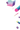# Introduction

Thinc is a lightweight deep learning library that offers an elegant, type-checked, functional-programming API for composing models, with support for layers defined in other frameworks such as PyTorch, TensorFlow or MXNet. You can use Thinc as an interface layer, a standalone toolkit or a flexible way to develop new models. Previous versions of Thinc have been running quietly in production in thousands of companies, via both spaCy and Prodigy. We wrote the new version to let users compose, configure and deploy custom models built with their favorite framework. The end result is a library quite different in its design, that’s easy to understand, plays well with others, and is a lot of fun to use.

with custom types and `mypy` plugin

``````from thinc.api import PyTorchWrapper, TensorFlowWrapper

pt_model = PyTorchWrapper(create_pytorch_model())
tf_model = TensorFlowWrapper(create_tensorflow_model())
# You can even stitch together strange hybrids
# (not efficient, but possible)
frankenmodel = chain(add(pt_model, tf_model), Linear(128), logistic())
``````
##### Wrap PyTorch, TensorFlow & MXNet models for use in your network

``````def CaptionRater(
text_encoder: Model[List[str], Floats2d],
image_encoder: Model[List[Path], Floats2d]
) -> Model[Tuple[List[str], List[Path]], Floats2d]:
return chain(
concatenate(
chain(get_item(0), text_encoder),
chain(get_item(1), image_encoder)
),
residual(Relu(nO=300, dropout=0.2, normalize=True)),
Softmax(2)
)
``````
##### Concise functional-programming approach to model definition

using composition rather than inheritance

``````apply_on = lambda layer, i: chain(getitem(i), layer)
with Model.define_operators({"^": apply_on, ">>": chain, "|": concatenate}):
model = (
(text_encoder ^ 0 | image_encoder ^ 1)
>> residual(Relu(nO=300, dropout=0.2, normalize=True)
>> Softmax(2)
)
``````

``````[optimizer]

[optimizer.learn_rate]
@schedules = "slanted_triangular.v1"
max_rate = 0.1
num_steps = 5000
``````
##### Integrated config system

to describe trees of objects and hyperparameters

``````from thinc.api import NumpyOps, set_current_ops

def CustomOps(NumpyOps):
def some_custom_op_my_layers_needs(...):
...
set_current_ops(CustomOps())
``````
##### Choice of extensible backends

``````encode_sentence = chain(
list2ragged(),  # concatenate sequences
with_array(  # ignore outer sequence structure (temporarily)
concatenate(Embed(128, column=0), Embed(128, column=1)),
Mish(128, dropout=0.2, normalize=True)
),
ParametricAttention(128),
reduce_mean()
)
``````
##### First-class support for variable-length sequences

multiple built-in sequence representations and your layers can use any object

``````for i in range(10):
for X, Y in train_batches:
Yh, backprop = model.begin_update(X)
loss, dYh = get_loss(Yh, Y)
backprop(dYh)
model.finish_update(optimizer)
``````

View more